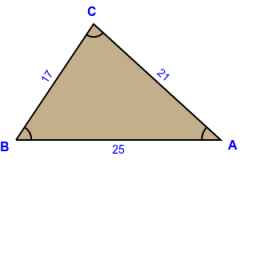# Loonie

Loonie has three wooden sticks measuring 17 inches, 21 inches, and 25 inches. He lays them down to form a triangle. Find the measure of the angle enclosed by 17 inches and 21 inches. (Express answers to the nearest hundredths) (using the law of cosines)

γ =  81.5435 °

### Step-by-step explanation:

Try calculation via our triangle calculator.Did you find an error or inaccuracy? Feel free to write us. Thank you!

Tips for related online calculators
Need help calculating sum, simplifying, or multiplying fractions? Try our fraction calculator.
Do you want to convert time units like minutes to seconds?
Cosine rule uses trigonometric SAS triangle calculator.
The Pythagorean theorem is the base for the right triangle calculator.